Courses

RD Sharma Solutions -Ex-17.1, Constructions, Class 9, Maths Notes | Study RD Sharma Solutions for Class 9 Mathematics - Class 9

Class 9: RD Sharma Solutions -Ex-17.1, Constructions, Class 9, Maths Notes | Study RD Sharma Solutions for Class 9 Mathematics - Class 9

The document RD Sharma Solutions -Ex-17.1, Constructions, Class 9, Maths Notes | Study RD Sharma Solutions for Class 9 Mathematics - Class 9 is a part of the Class 9 Course RD Sharma Solutions for Class 9 Mathematics.
All you need of Class 9 at this link: Class 9

Q1. Draw a line segment of length 8.6cm. Bisect it and measure the length of each part.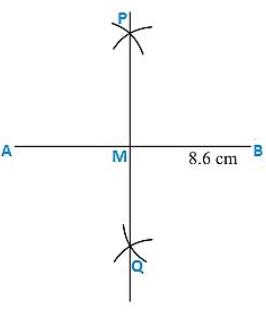Steps of construction:

1. Draw a line segment AB of 8.6cm.
2. Keeping A as center and radius more than half of AB draw arcs on each side of AB.
3. Keeping B as center and the same radius draw arcs on each side of AB cutting the previous arcs at P and Q respectively.
4. Join the points P and Q which intersects AB at C.

Therefore AC= BC= 4.3cm

Q2. Draw a line segment AB of length 5.8cm. Draw the perpendicular bisector of this line segment.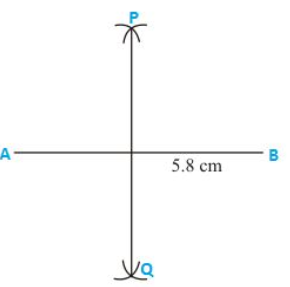Steps of construction:

1. Draw a line segment AB of 5.8cm.
2. Keeping A as center and radius more than half of AB draw arcs on each side of AB.
3. Keeping B as center and the same radius draw arcs on each side of AB cutting the previous arcs at P and Q respectively.
4. Join the points P and Q.

Hence PQ is the perpendicular bisector of AB

Q3. Draw a circle with center at point O and radius 5cm. Draw its chord AB, the perpendicular bisector of line segment AB. Does it pass through the center of the circle?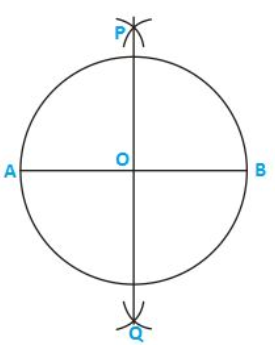Steps of construction:

1. Keeping O as center and radius as 5cm draw a circle.
2. Draw a chord AB.
3. Keeping A as center and radius more than half of AB draw arcs one on each side of chord AB.
4. Keeping B as center and the same radius draw arcs cutting the previous arcs at points P and Q respectively.
5. Join the points P and Q.

Therefore the perpendicular bisector PQ of chord AB passes through the center of the circle.

Q4. Draw a circle with center at point O. Draw its two chords AB and CD such that AB is not parallel to CD. Draw the perpendicular bisectors of AB and CD. At what point do they intersect?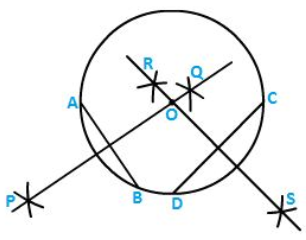Steps of construction:

1. Keeping O as the centre and any radius draw a circle.
2. Draw two chords AB and CD.
3. Keeping A as center and radius more than half of AB draw arcs, one on each side of AB.
4. Keeping B as center and the same radius draw arcs cutting the previous arcs at point P and Q respectively.
5. Join the points P and Q.
6. Keeping D as center and radius more than half of DC draw arcs, one on each side of DC.
7. Keeping C as center and the same radius draw arcs cutting the previous arcs at points R and S respectively.
8. Join the points R and S.

Both the perpendicular bisectors PQ and RS intersect each other at the center O of the circle.

Q5. Draw a line segment of length 10cm and bisect it. Further, bisect one of the equal parts and measure its length.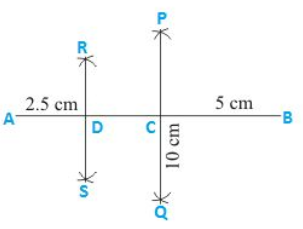Steps of construction:

1. Draw a line segment AB of length 10cms.
2. Keeping A as center and radius more than half of AB draw arcs one on each side of AB.
3. Keeping B as center and same radius draw arcs cutting the previous arc at point P and Q respectively.
4. Join P and Q which intersects AB at C.
5. Keeping A as center and radius more than half of AC draw arcs on each side of AC.
6. Keeping C as center and the same radius draw arcs cutting the previous arcs at points R and S respectively.
7. Join points R and S which intersects AC at D.

Q6. Draw a line segment AB bisect it. Bisect one of the equal parts to obtain a line segment of length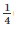(AB).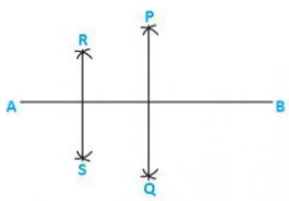Steps of construction:

1. Draw a line segment AB.
2. With A as center and radius more than half of AB draw arcs, one on each side of AB.
3. With B as the center and same radius draw arcs cutting the previous arcs at points P and Q respectively.
4. Join P and Q which intersects AB at C.
5. With A as center and radius more than half of AC draw arcs, one on each side of AC.
6. With C as the center and same radius draw arcs cutting the previous arcs at R and S respectively.
7. Join points R and S which intersects AC at D.

Therefore AD=(AB)

Q7. Draw a line segment AB and by ruler and compasses, obtain a line segment of length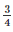(AB).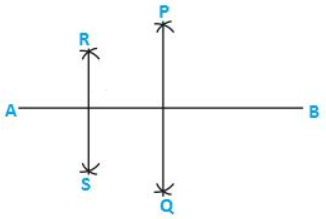Steps of construction:

1. Draw a line segment AB.
2. With A as center and radius more than half of AB draw arcs, one on each side of AB.
3. With B as the center and same radius draw arcs cutting the previous arcs at points P and Q respectively.
4. Join P and Q which intersects AB at C.
5. With A as center and radius more than half of AC draw arcs, one on each side of AC.
6. With C as the center and same radius draw arcs cutting the previous arcs at R and S respectively.
7. Join points R and S which intersects AC at D.

Therefore AD=((AB)

The document RD Sharma Solutions -Ex-17.1, Constructions, Class 9, Maths Notes | Study RD Sharma Solutions for Class 9 Mathematics - Class 9 is a part of the Class 9 Course RD Sharma Solutions for Class 9 Mathematics.
All you need of Class 9 at this link: Class 9Use Code STAYHOME200 and get INR 200 additional OFF Use Coupon Code

Top Courses for Class 9RD Sharma Solutions for Class 9 Mathematics

91 docs

Top Courses for Class 9Track your progress, build streaks, highlight & save important lessons and more!

,

,

,

,

,

,

,

,

,

,

,

,

,

,

,

,

,

,

,

,

,

,

,

,

,

,

,

,

,

,

;